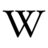# EccentricityThis article is based on a Wikipedia article prior to 15 June 2009 and is controlled by version 1.2 of the the GNU Free Documentation License (GFDL).

## Definition

Any orbit in planetary dynamics can be assumed to be of conic cross-section shape. The eccentricity of this conic section, the orbit's eccentricity, is an important parameter of the orbit that defines its absolute shape. Eccentricity may be interpreted as a measure of how much this shape deviates from a circle.

Eccentricity ($e\,\!$) is strictly defined for all circular, elliptic, parabolic and hyperbolic orbits and may take following values:

• for circular orbits: $e=0\,\!$,
• for elliptic orbits: $0,
• for parabolic orbits: $e=1\,\!$,
• for hyperbolic orbits: $e>1\,\!$.

## Calculation

For elliptic orbits, eccentricity can be calculated from distance at periapsis and apoapsis:

$e={{d_{a}-d_{p}} \over {d_{a}+d_{p}}}$$=1-{\frac {2}{(d_{a}/d_{p})+1}}$where:

• $d_{p}\,\!$is distance at periapsis (closest approach),
• $d_{a}\,\!$is distance at apoapsis (farthest approach).